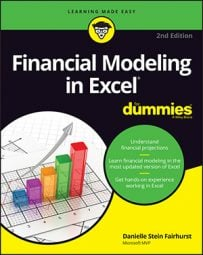##### Financial Modeling in Excel For DummiesIf you’re getting a financial model audited by a third party, one of the many things they’ll check for is the number of unique formulas in the model. A good modeler will create as few unique formulas as possible as he’s building a model.

In the following example, the modeler is calculating portfolio returns of different amounts. The image shows the formulas, rather than the calculated values. The way this block has been created in this instance, nine different formulas have been used, which is a very slow and inefficient way of creating this calculation block.

Download File 0401.xlsx and select the tab labeled 4-7 or simply open Excel, re-create this example and try it out for yourself. You’ll see that creating the formulas is a slow and tedious process.

If the formulas aren’t all showing when you download and open this file, click the Show Formulas button in the Auditing group on the Formulas tab in the Ribbon. Or, you can use the Ctrl+` shortcut (the ` symbol can be found to the left of the 1 on most keyboards, under the ~ sign).

The task is made much simpler by merely including a few dollar signs in the formula in cell B2 and then copying. Using one single formula and then copying it across and down the calculation block is much quicker and less prone to error.

Using mixed referencing within the formula — by putting a dollar sign before the row or column to be anchored — is a far more efficient way of modeling.

This concept of building your models with consistent formulas wherever possible saves time, avoids error, and is much easier to audit. And it’s a key component of good financial modeling. If you only pick up one modeling technique from this book, this is it!

Modelers should strive for consistency of formulas within models. But consistency, in general, is something to aim for in all aspects of the model build. Use consistent colors and formatting, consistent labels, and even consistent layouts. If sheets are similar, work on the sheet until you’re completely happy with the layout, and then copy the sheet.

This way, the design, formatting, and layout will be identical. Then when you need to update it, group the sheets to make global changes. Have columns and rows matching on multiple sheets — for example, on calculation sheets, if January’s calculations start in column F, then January should always start in column F on every calculation sheet.